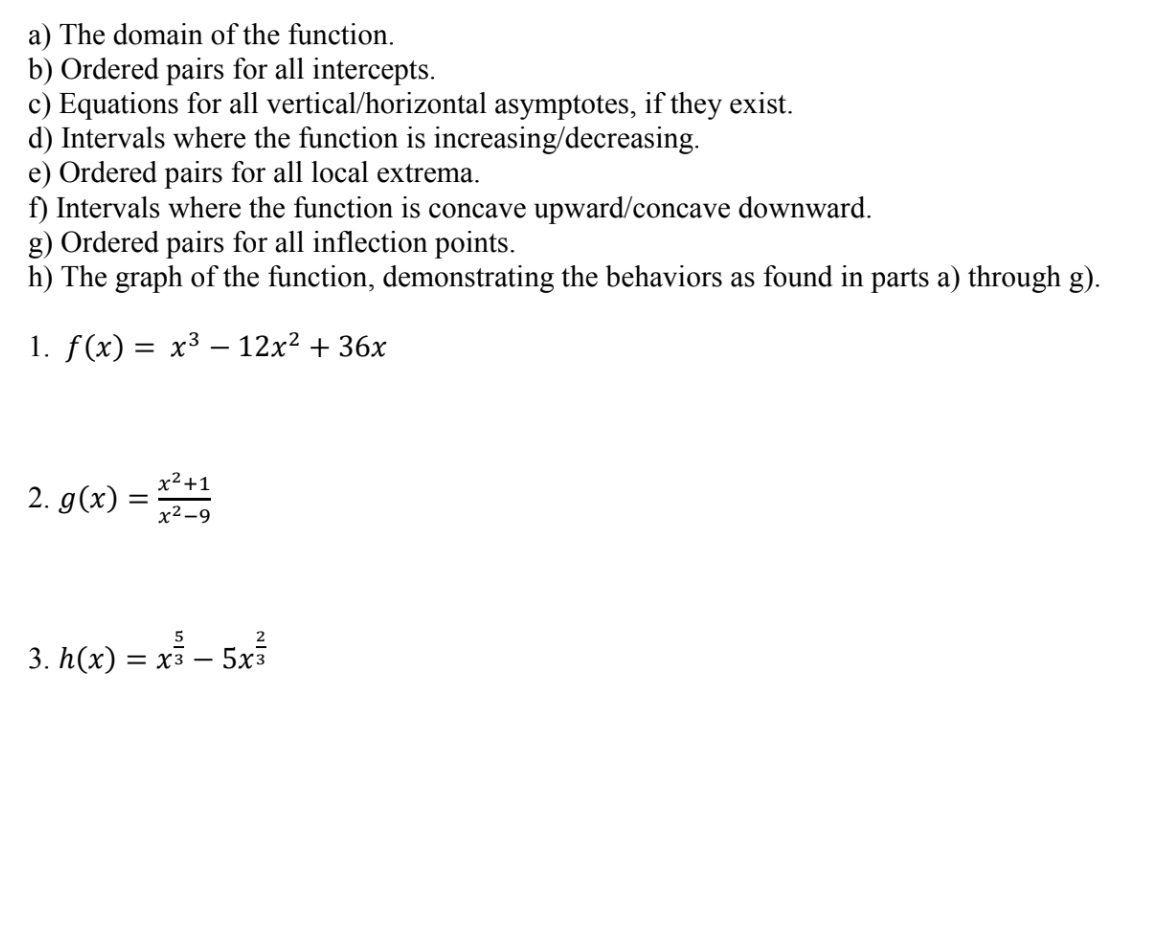# a) The domain of the function.b) Ordered pairs for all intercepts.c) Equations for all vertical/horizontal asymptotes, if they existd) Intervals where the function is increasing/decreasinge) Ordered pairs for all local extremaft) Intervals where the function is concave upward/concave downwardg) Ordered pairs for all inflection points.h) The graph of the function, demonstrating the behaviors as found in parts a) through g)I. f(x)-x3-12x2 + 36X2x2-9523. h(x) -xs - 5x3

Question
26 views

Solve q#2 from d to hhelp_outlineImage Transcriptionclosea) The domain of the function. b) Ordered pairs for all intercepts. c) Equations for all vertical/horizontal asymptotes, if they exist d) Intervals where the function is increasing/decreasing e) Ordered pairs for all local extrema ft) Intervals where the function is concave upward/concave downward g) Ordered pairs for all inflection points. h) The graph of the function, demonstrating the behaviors as found in parts a) through g) I. f(x)-x3-12x2 + 3 6X 2 x2-9 5 2 3. h(x) -xs - 5x3 fullscreen
check_circle

Step 1

Hello there! I see that there are more than 3 sub parts in the questin. According to our policies cannot answer more than 3 sub parts. Please do find the solution for d,e,f for question 2. If you have any doubts in remaining parts kindly make a new request.

Step 2

Given g(x) = (x2+1)/(x2-9)

A function is said to be increasing n certain interval, if its derivative is greater than 0 in that paticular interval. If the derivative is less than 0 in an interval then it is said to be decreasng in that particular interval.

For given function g(x), its derivative is calculated as shown below. We get                               g'(x) = -16x/(x2 -9)2

Here we can see that this function is not defined when x= 3, x = -3 as the denominator will be 0. Clearly we can see that for all negative values the value of g'(x) will be positive and greater than 0 and for all positive x values it will be less than 0. So we say for all negative values the function g(x) is increasing except for x = - 3 and for all positive values the function g(x) is decreasing except x = 3.

Interval where g(x) is  increasing (-∞ , -3) U ( -3, 0)

Interval where g(x) is  decreasing (0 , 3) U  (3 , ∞)

Step 3

The ordered pairs for local extrema can be found out when we equatio g'(x) = 0. Equating g'(x) to 0 we get the following as shown below. So for only x = 0 the g'(x) w...

### Want to see the full answer?

See Solution

#### Want to see this answer and more?

Solutions are written by subject experts who are available 24/7. Questions are typically answered within 1 hour.*

See Solution
*Response times may vary by subject and question.
Tagged in

### Derivative